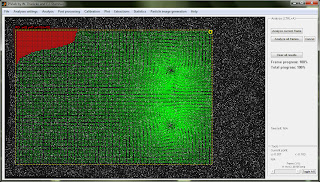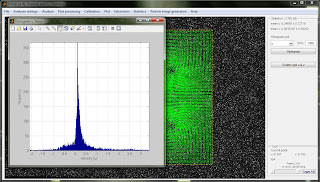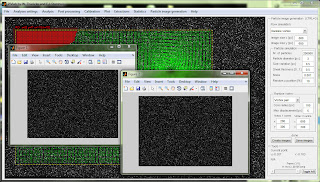### ScreenshotsImport image promptPIV settingsAnalyzed image pair, region of interest and image maskVector validationDerived parameters: velocity magnitudeStreamlinesMeasuring angles and distancesExtracting data along a polyline (u velocity component)Extracting data along polyline (vorticity)Extracting data along circular path (tangential velocity)Extracting data along a series of circular paths and integrating tangential velocity (= vortex circulation)Extracting mean velocity magnitude in specified areaVorticity area integral for a series of circular areas (= vortex circulation)u velocity component histogramGeneration of synthetic particle images for PIV analyses